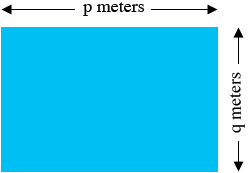SEARCH HOMEMath Central Quandaries & QueriesQuestion from Allie: The length of a rectangular swimming pool is p m and its width is q m. Its given that the length of the swimming pool is twice its with. if the perimeter of the swimming pool is 150 m, find the values of p and qHi Allie,

Sometimes it helps to draw a rough sketch.In this problem you are told that the length of the pool is $p$ meters and its width is $q$ meters. You are also told that the length is twice the width so

$p = 2 q.$

You are also told that the perimeter is 150 meters. What is the perimeter in terms of $p$ and $q?$ This expression is equal to 150 meters so now you have two linear equations in $p$ and $q.$ Solve this pair of equations for $p$ and $q.$